# 一份不可多得的 TS 学习指南（1.8W字）

197122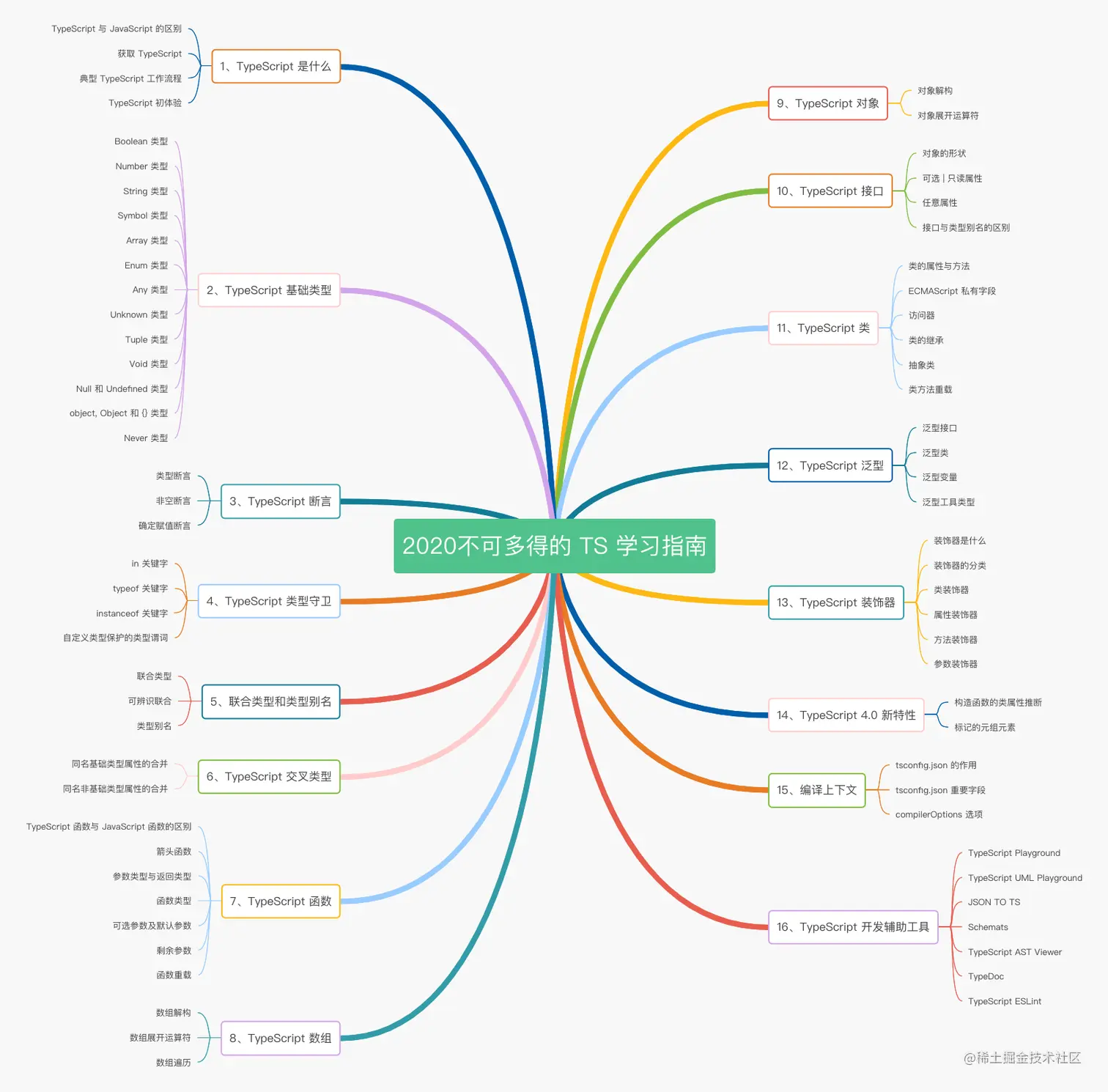### 一、TypeScript 是什么

TypeScript 是一种由微软开发的自由和开源的编程语言。它是 JavaScript 的一个超集，而且本质上向这个语言添加了可选的静态类型和基于类的面向对象编程。

TypeScript 提供最新的和不断发展的 JavaScript 特性，包括那些来自 2015 年的 ECMAScript 和未来的提案中的特性，比如异步功能和 Decorators，以帮助建立健壮的组件。下图显示了 TypeScript 与 ES5、ES2015 和 ES2016 之间的关系：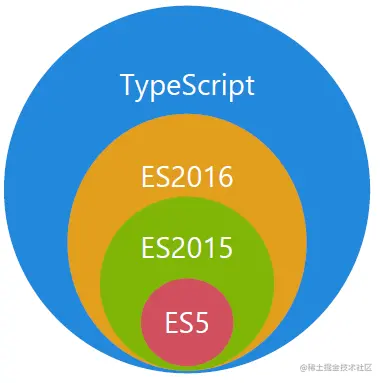#### 1.1 TypeScript 与 JavaScript 的区别

TypeScriptJavaScript
JavaScript 的超集用于解决大型项目的代码复杂性一种脚本语言，用于创建动态网页

#### 1.2 获取 TypeScript

##### 1.安装 TypeScript
``````\$ npm install -g typescript
``````
##### 2.验证 TypeScript
``````\$ tsc -v
# Version 4.0.2
``````
##### 3.编译 TypeScript 文件
``````\$ tsc helloworld.ts
# helloworld.ts => helloworld.js
``````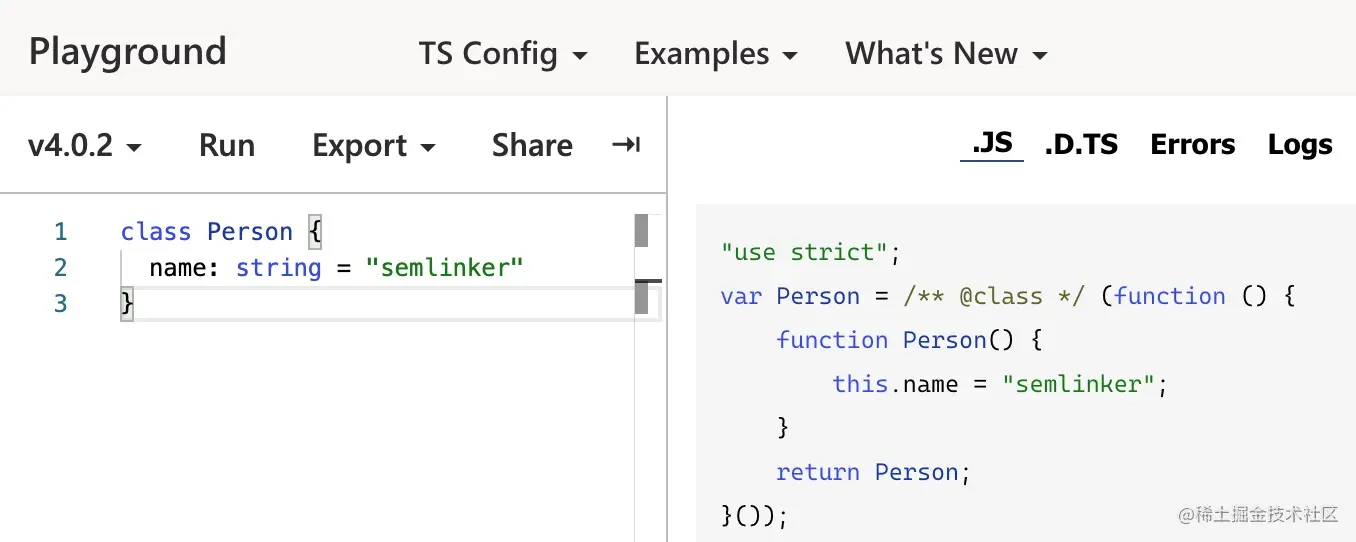#### 1.3 典型 TypeScript 工作流程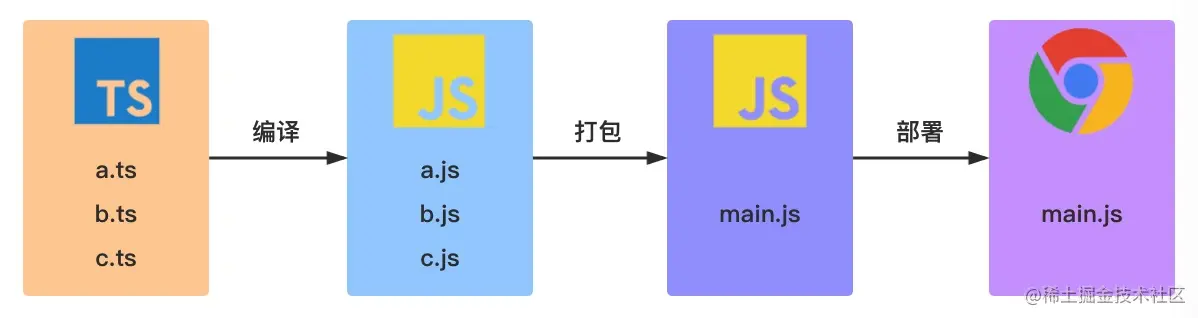#### 1.4 TypeScript 初体验

``````function greet(person: string) {
return 'Hello, ' + person;
}

console.log(greet("TypeScript"));
``````

``````"use strict";
function greet(person) {
return 'Hello, ' + person;
}
console.log(greet("TypeScript"));
``````

### 二、TypeScript 基础类型

#### 2.1 Boolean 类型

``````let isDone: boolean = false;
// ES5：var isDone = false;
``````

#### 2.2 Number 类型

``````let count: number = 10;
// ES5：var count = 10;
``````

#### 2.3 String 类型

``````let name: string = "semliker";
``````

#### 2.4 Symbol 类型

``````const sym = Symbol();
let obj = {
};

``````

#### 2.5 Array 类型

``````let list: number[] = [1, 2, 3];
// ES5：var list = [1,2,3];

let list: Array<number> = [1, 2, 3]; // Array<number>泛型语法
// ES5：var list = [1,2,3];
``````

#### 2.6 Enum 类型

##### 1.数字枚举
``````enum Direction {
NORTH,
SOUTH,
EAST,
WEST,
}

let dir: Direction = Direction.NORTH;
``````

``````"use strict";
var Direction;
(function (Direction) {
Direction[(Direction["NORTH"] = 0)] = "NORTH";
Direction[(Direction["SOUTH"] = 1)] = "SOUTH";
Direction[(Direction["EAST"] = 2)] = "EAST";
Direction[(Direction["WEST"] = 3)] = "WEST";
})(Direction || (Direction = {}));
var dir = Direction.NORTH;
``````

``````enum Direction {
NORTH = 3,
SOUTH,
EAST,
WEST,
}
``````
##### 2.字符串枚举

``````enum Direction {
NORTH = "NORTH",
SOUTH = "SOUTH",
EAST = "EAST",
WEST = "WEST",
}
``````

``````"use strict";
var Direction;
(function (Direction) {
Direction["NORTH"] = "NORTH";
Direction["SOUTH"] = "SOUTH";
Direction["EAST"] = "EAST";
Direction["WEST"] = "WEST";
})(Direction || (Direction = {}));
``````

``````enum Direction {
NORTH,
SOUTH,
EAST,
WEST,
}

let dirName = Direction; // NORTH
let dirVal = Direction["NORTH"]; // 0
``````

##### 3.常量枚举

``````const enum Direction {
NORTH,
SOUTH,
EAST,
WEST,
}

let dir: Direction = Direction.NORTH;
``````

``````"use strict";
var dir = 0 /* NORTH */;
``````
##### 4.异构枚举

``````enum Enum {
A,
B,
C = "C",
D = "D",
E = 8,
F,
}
``````

``````"use strict";
var Enum;
(function (Enum) {
Enum[Enum["A"] = 0] = "A";
Enum[Enum["B"] = 1] = "B";
Enum["C"] = "C";
Enum["D"] = "D";
Enum[Enum["E"] = 8] = "E";
Enum[Enum["F"] = 9] = "F";
})(Enum || (Enum = {}));
``````

``````console.log(Enum.A) //输出：0
console.log(Enum) // 输出：A
``````

#### 2.7 Any 类型

``````let notSure: any = 666;
notSure = false;
``````

`any` 类型本质上是类型系统的一个逃逸舱。作为开发者，这给了我们很大的自由：TypeScript 允许我们对 `any` 类型的值执行任何操作，而无需事先执行任何形式的检查。比如：

``````let value: any;

value.foo.bar; // OK
value.trim(); // OK
value(); // OK
new value(); // OK
value; // OK
``````

#### 2.8 Unknown 类型

``````let value: unknown;

value = true; // OK
value = 42; // OK
value = "Hello World"; // OK
value = []; // OK
value = {}; // OK
value = Math.random; // OK
value = null; // OK
value = undefined; // OK
value = new TypeError(); // OK
value = Symbol("type"); // OK
``````

`value` 变量的所有赋值都被认为是类型正确的。但是，当我们尝试将类型为 `unknown` 的值赋值给其他类型的变量时会发生什么？

``````let value: unknown;

let value1: unknown = value; // OK
let value2: any = value; // OK
let value3: boolean = value; // Error
let value4: number = value; // Error
let value5: string = value; // Error
let value6: object = value; // Error
let value7: any[] = value; // Error
let value8: Function = value; // Error
``````

`unknown` 类型只能被赋值给 `any` 类型和 `unknown` 类型本身。直观地说，这是有道理的：只有能够保存任意类型值的容器才能保存 `unknown` 类型的值。毕竟我们不知道变量 `value` 中存储了什么类型的值。

``````let value: unknown;

value.foo.bar; // Error
value.trim(); // Error
value(); // Error
new value(); // Error
value; // Error
``````

`value` 变量类型设置为 `unknown` 后，这些操作都不再被认为是类型正确的。通过将 `any` 类型改变为 `unknown` 类型，我们已将允许所有更改的默认设置，更改为禁止任何更改。

#### 2.9 Tuple 类型

``````let tupleType: [string, boolean];
``````

``````console.log(tupleType); // semlinker
console.log(tupleType); // true
``````

``````tupleType = [true, "semlinker"];
``````

``````: Type 'true' is not assignable to type 'string'.
: Type 'string' is not assignable to type 'boolean'.
``````

``````tupleType = ["semlinker"];
``````

``````Property '1' is missing in type '[string]' but required in type '[string, boolean]'.
``````

#### 2.10 Void 类型

``````// 声明函数返回值为void
function warnUser(): void {
console.log("This is my warning message");
}
``````

``````"use strict";
function warnUser() {
console.log("This is my warning message");
}
``````

``````let unusable: void = undefined;
``````

#### 2.11 Null 和 Undefined 类型

TypeScript 里，`undefined``null` 两者有各自的类型分别为 `undefined``null`

``````let u: undefined = undefined;
let n: null = null;
``````

#### 2.12 object, Object 和 {} 类型

##### 1.object 类型

object 类型是：TypeScript 2.2 引入的新类型，它用于表示非原始类型。

``````// node_modules/typescript/lib/lib.es5.d.ts
interface ObjectConstructor {
create(o: object | null): any;
// ...
}

const proto = {};

Object.create(proto);     // OK
Object.create(null);      // OK
Object.create(undefined); // Error
Object.create(1337);      // Error
Object.create(true);      // Error
Object.create("oops");    // Error
``````
##### 2.Object 类型

Object 类型：它是所有 Object 类的实例的类型，它由以下两个接口来定义：

• Object 接口定义了 Object.prototype 原型对象上的属性；
``````// node_modules/typescript/lib/lib.es5.d.ts
interface Object {
constructor: Function;
toString(): string;
toLocaleString(): string;
valueOf(): Object;
hasOwnProperty(v: PropertyKey): boolean;
isPrototypeOf(v: Object): boolean;
propertyIsEnumerable(v: PropertyKey): boolean;
}
``````
• ObjectConstructor 接口定义了 Object 类的属性。
``````// node_modules/typescript/lib/lib.es5.d.ts
interface ObjectConstructor {
/** Invocation via `new` */
new(value?: any): Object;
/** Invocation via function calls */
(value?: any): any;
getPrototypeOf(o: any): any;
// ···
}

declare var Object: ObjectConstructor;
``````

Object 类的所有实例都继承了 Object 接口中的所有属性。

##### 3.{} 类型

{} 类型描述了一个没有成员的对象。当你试图访问这样一个对象的任意属性时，TypeScript 会产生一个编译时错误。

``````// Type {}
const obj = {};

// Error: Property 'prop' does not exist on type '{}'.
``````

``````// Type {}
const obj = {};

// "[object Object]"
obj.toString();
``````

#### 2.13 Never 类型

`never` 类型表示的是那些永不存在的值的类型。 例如，`never` 类型是那些总是会抛出异常或根本就不会有返回值的函数表达式或箭头函数表达式的返回值类型。

``````// 返回never的函数必须存在无法达到的终点
function error(message: string): never {
throw new Error(message);
}

function infiniteLoop(): never {
while (true) {}
}
``````

``````type Foo = string | number;

function controlFlowAnalysisWithNever(foo: Foo) {
if (typeof foo === "string") {
// 这里 foo 被收窄为 string 类型
} else if (typeof foo === "number") {
// 这里 foo 被收窄为 number 类型
} else {
// foo 在这里是 never
const check: never = foo;
}
}
``````

``````type Foo = string | number | boolean;
``````

`controlFlowAnalysisWithNever` 方法总是穷尽了 Foo 的所有可能类型。 通过这个示例，我们可以得出一个结论：使用 never 避免出现新增了联合类型没有对应的实现，目的就是写出类型绝对安全的代码。

### 三、TypeScript 断言

#### 3.1 类型断言

##### 1.“尖括号” 语法
``````let someValue: any = "this is a string";
let strLength: number = (<string>someValue).length;
``````
##### 2.as 语法
``````let someValue: any = "this is a string";
let strLength: number = (someValue as string).length;
``````

#### 3.2 非空断言

##### 1.忽略 undefined 和 null 类型
``````function myFunc(maybeString: string | undefined | null) {
// Type 'string | null | undefined' is not assignable to type 'string'.
// Type 'undefined' is not assignable to type 'string'.
const onlyString: string = maybeString; // Error
const ignoreUndefinedAndNull: string = maybeString!; // Ok
}
``````
##### 2.调用函数时忽略 undefined 类型
``````type NumGenerator = () => number;

function myFunc(numGenerator: NumGenerator | undefined) {
// Object is possibly 'undefined'.(2532)
// Cannot invoke an object which is possibly 'undefined'.(2722)
const num1 = numGenerator(); // Error
const num2 = numGenerator!(); //OK
}
``````

``````const a: number | undefined = undefined;
const b: number = a!;
console.log(b);
``````

``````"use strict";
const a = undefined;
const b = a;
console.log(b);
``````

#### 3.3 确定赋值断言

``````let x: number;
initialize();
// Variable 'x' is used before being assigned.(2454)
console.log(2 * x); // Error

function initialize() {
x = 10;
}
``````

``````let x!: number;
initialize();
console.log(2 * x); // Ok

function initialize() {
x = 10;
}
``````

### 四、类型守卫

#### 4.1 in 关键字

``````interface Admin {
name: string;
privileges: string[];
}

interface Employee {
name: string;
startDate: Date;
}

type UnknownEmployee = Employee | Admin;

function printEmployeeInformation(emp: UnknownEmployee) {
console.log("Name: " + emp.name);
if ("privileges" in emp) {
console.log("Privileges: " + emp.privileges);
}
if ("startDate" in emp) {
console.log("Start Date: " + emp.startDate);
}
}
``````

#### 4.2 typeof 关键字

``````function padLeft(value: string, padding: string | number) {
if (typeof padding === "number") {
return Array(padding + 1).join(" ") + value;
}
if (typeof padding === "string") {
}
throw new Error(`Expected string or number, got '\${padding}'.`);
}
``````

`typeof` 类型保护只支持两种形式：`typeof v === "typename"``typeof v !== typename``"typename"` 必须是 `"number"``"string"``"boolean"``"symbol"`。 但是 TypeScript 并不会阻止你与其它字符串比较，语言不会把那些表达式识别为类型保护。

#### 4.3 instanceof 关键字

``````interface Padder {
}

constructor(private numSpaces: number) {}
return Array(this.numSpaces + 1).join(" ");
}
}

constructor(private value: string) {}
return this.value;
}
}

}
``````

#### 4.4 自定义类型保护的类型谓词

``````function isNumber(x: any): x is number {
return typeof x === "number";
}

function isString(x: any): x is string {
return typeof x === "string";
}
``````

### 五、联合类型和类型别名

#### 5.1 联合类型

``````const sayHello = (name: string | undefined) => {
/* ... */
};
``````

``````sayHello("semlinker");
sayHello(undefined);
``````

``````let num: 1 | 2 = 1;
type EventNames = 'click' | 'scroll' | 'mousemove';
``````

#### 5.2 可辨识联合

TypeScript 可辨识联合（Discriminated Unions）类型，也称为代数数据类型或标签联合类型。它包含 3 个要点：可辨识、联合类型和类型守卫。

##### 1.可辨识

``````enum CarTransmission {
Automatic = 200,
Manual = 300
}

interface Motorcycle {
vType: "motorcycle"; // discriminant
make: number; // year
}

interface Car {
vType: "car"; // discriminant
transmission: CarTransmission
}

interface Truck {
vType: "truck"; // discriminant
capacity: number; // in tons
}
``````

##### 2.联合类型

``````type Vehicle = Motorcycle | Car | Truck;
``````

##### 3.类型守卫

``````const EVALUATION_FACTOR = Math.PI;

function evaluatePrice(vehicle: Vehicle) {
return vehicle.capacity * EVALUATION_FACTOR;
}

const myTruck: Truck = { vType: "truck", capacity: 9.5 };
evaluatePrice(myTruck);
``````

``````Property 'capacity' does not exist on type 'Vehicle'.
Property 'capacity' does not exist on type 'Motorcycle'.
``````

``````function evaluatePrice(vehicle: Vehicle) {
switch(vehicle.vType) {
case "car":
return vehicle.transmission * EVALUATION_FACTOR;
case "truck":
return vehicle.capacity * EVALUATION_FACTOR;
case "motorcycle":
return vehicle.make * EVALUATION_FACTOR;
}
}
``````

#### 5.3 类型别名

``````type Message = string | string[];

let greet = (message: Message) => {
// ...
};
``````

### 六、交叉类型

``````type PartialPointX = { x: number; };
type Point = PartialPointX & { y: number; };

let point: Point = {
x: 1,
y: 1
}
``````

#### 6.1 同名基础类型属性的合并

``````interface X {
c: string;
d: string;
}

interface Y {
c: number;
e: string
}

type XY = X & Y;
type YX = Y & X;

let p: XY;
let q: YX;
``````

``````p = { c: 6, d: "d", e: "e" };
``````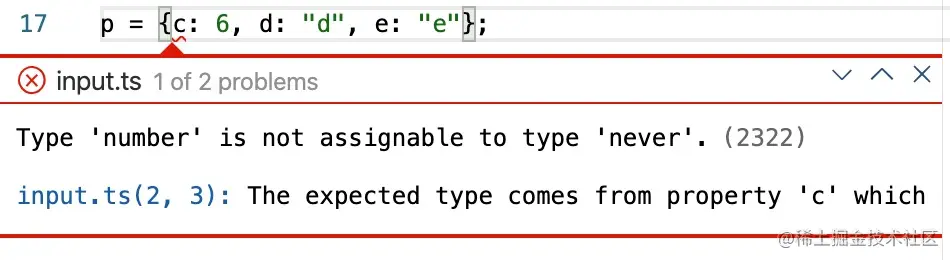``````q = { c: "c", d: "d", e: "e" };
``````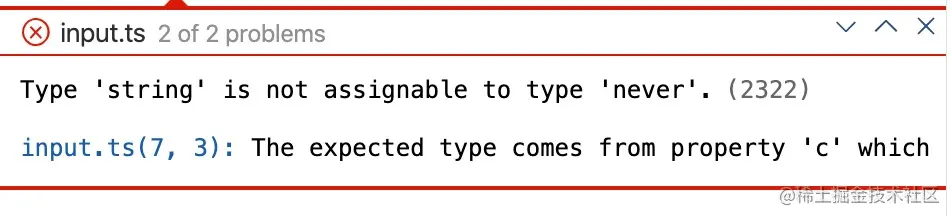#### 6.2 同名非基础类型属性的合并

``````interface D { d: boolean; }
interface E { e: string; }
interface F { f: number; }

interface A { x: D; }
interface B { x: E; }
interface C { x: F; }

type ABC = A & B & C;

let abc: ABC = {
x: {
d: true,
f: 666
}
};

console.log('abc:', abc);
``````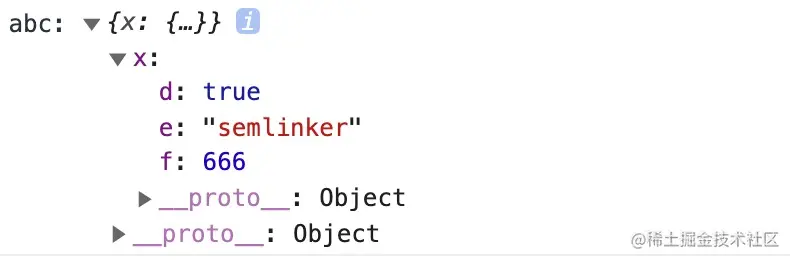### 七、TypeScript 函数

#### 7.1 TypeScript 函数与 JavaScript 函数的区别

TypeScriptJavaScript

#### 7.2 箭头函数

##### 1.常见语法
``````myBooks.forEach(() => console.log('reading'));

myBooks.forEach(title => console.log(title));

myBooks.forEach((title, idx, arr) =>
console.log(idx + '-' + title);
);

myBooks.forEach((title, idx, arr) => {
console.log(idx + '-' + title);
});
``````
##### 2.使用示例
``````// 未使用箭头函数
function Book() {
let self = this;
self.publishDate = 2016;
setInterval(function () {
console.log(self.publishDate);
}, 1000);
}

// 使用箭头函数
function Book() {
this.publishDate = 2016;
setInterval(() => {
console.log(this.publishDate);
}, 1000);
}
``````

#### 7.3 参数类型和返回类型

``````function createUserId(name: string, id: number): string {
return name + id;
}
``````

#### 7.4 函数类型

``````let IdGenerator: (chars: string, nums: number) => string;

function createUserId(name: string, id: number): string {
return name + id;
}

IdGenerator = createUserId;
``````

#### 7.5 可选参数及默认参数

``````// 可选参数
function createUserId(name: string, id: number, age?: number): string {
return name + id;
}

// 默认参数
function createUserId(
id: number,
age?: number
): string {
return name + id;
}
``````

#### 7.6 剩余参数

``````function push(array, ...items) {
items.forEach(function (item) {
array.push(item);
});
}

let a = [];
push(a, 1, 2, 3);
``````

#### 7.7 函数重载

``````function add(a: number, b: number): number;
function add(a: string, b: string): string;
function add(a: string, b: number): string;
function add(a: number, b: string): string;
function add(a: Combinable, b: Combinable) {
// type Combinable = string | number;
if (typeof a === 'string' || typeof b === 'string') {
return a.toString() + b.toString();
}
return a + b;
}
``````

``````class Calculator {
if (typeof a === 'string' || typeof b === 'string') {
return a.toString() + b.toString();
}
return a + b;
}
}

const calculator = new Calculator();
``````

### 八、TypeScript 数组

#### 8.1 数组解构

``````let x: number; let y: number; let z: number;
let five_array = [0,1,2,3,4];
[x,y,z] = five_array;
``````

#### 8.2 数组展开运算符

``````let two_array = [0, 1];
let five_array = [...two_array, 2, 3, 4];
``````

#### 8.3 数组遍历

``````let colors: string[] = ["red", "green", "blue"];
for (let i of colors) {
console.log(i);
}
``````

### 九、TypeScript 对象

#### 9.1 对象解构

``````let person = {
gender: "Male",
};

let { name, gender } = person;
``````

#### 9.2 对象展开运算符

``````let person = {
gender: "Male",
};

// 组装对象
let personWithAge = { ...person, age: 33 };

// 获取除了某些项外的其它项
let { name, ...rest } = person;
``````

### 十、TypeScript 接口

TypeScript 中的接口是一个非常灵活的概念，除了可用于对类的一部分行为进行抽象以外，也常用于对「对象的形状（Shape）」进行描述。

#### 10.1 对象的形状

``````interface Person {
name: string;
age: number;
}

age: 33,
};
``````

#### 10.2 可选 | 只读属性

``````interface Person {
age?: number;
}
``````

``````let a: number[] = [1, 2, 3, 4];
ro = 12; // error!
ro.push(5); // error!
ro.length = 100; // error!
a = ro; // error!
``````

#### 10.3 任意属性

``````interface Person {
name: string;
age?: number;
[propName: string]: any;
}

const p1 = { name: "semlinker" };
const p2 = { name: "lolo", age: 5 };
const p3 = { name: "kakuqo", sex: 1 }
``````

#### 10.4 接口与类型别名的区别

##### 1.Objects/Functions

``````interface Point {
x: number;
y: number;
}

interface SetPoint {
(x: number, y: number): void;
}
``````

``````type Point = {
x: number;
y: number;
};

type SetPoint = (x: number, y: number) => void;
``````
##### 2.Other Types

``````// primitive
type Name = string;

// object
type PartialPointX = { x: number; };
type PartialPointY = { y: number; };

// union
type PartialPoint = PartialPointX | PartialPointY;

// tuple
type Data = [number, string];
``````
##### 3.Extend

Interface extends interface

``````interface PartialPointX { x: number; }
interface Point extends PartialPointX {
y: number;
}
``````

Type alias extends type alias

``````type PartialPointX = { x: number; };
type Point = PartialPointX & { y: number; };
``````

Interface extends type alias

``````type PartialPointX = { x: number; };
interface Point extends PartialPointX { y: number; }
``````

Type alias extends interface

``````interface PartialPointX { x: number; }
type Point = PartialPointX & { y: number; };
``````
##### 4.Implements

``````interface Point {
x: number;
y: number;
}

class SomePoint implements Point {
x = 1;
y = 2;
}

type Point2 = {
x: number;
y: number;
};

class SomePoint2 implements Point2 {
x = 1;
y = 2;
}

type PartialPoint = { x: number; } | { y: number; };

// A class can only implement an object type or
// intersection of object types with statically known members.
class SomePartialPoint implements PartialPoint { // Error
x = 1;
y = 2;
}
``````
##### 5.Declaration merging

``````interface Point { x: number; }
interface Point { y: number; }

const point: Point = { x: 1, y: 2 };
``````

### 十一、TypeScript 类

#### 11.1 类的属性与方法

``````class Greeter {
// 静态属性
static cname: string = "Greeter";
// 成员属性
greeting: string;

// 构造函数 - 执行初始化操作
constructor(message: string) {
this.greeting = message;
}

// 静态方法
static getClassName() {
return "Class name is Greeter";
}

// 成员方法
greet() {
return "Hello, " + this.greeting;
}
}

let greeter = new Greeter("world");
``````

``````"use strict";
var Greeter = /** @class */ (function () {
// 构造函数 - 执行初始化操作
function Greeter(message) {
this.greeting = message;
}
// 静态方法
Greeter.getClassName = function () {
return "Class name is Greeter";
};
// 成员方法
Greeter.prototype.greet = function () {
return "Hello, " + this.greeting;
};
// 静态属性
Greeter.cname = "Greeter";
return Greeter;
}());
var greeter = new Greeter("world");
``````

#### 11.2 ECMAScript 私有字段

``````class Person {
#name: string;

constructor(name: string) {
this.#name = name;
}

greet() {
console.log(`Hello, my name is \${this.#name}!`);
}
}

//     ~~~~~
// Property '#name' is not accessible outside class 'Person'
// because it has a private identifier.
``````

• 私有字段以 `#` 字符开头，有时我们称之为私有名称；
• 每个私有字段名称都唯一地限定于其包含的类；
• 不能在私有字段上使用 TypeScript 可访问性修饰符（如 public 或 private）；
• 私有字段不能在包含的类之外访问，甚至不能被检测到。

#### 11.3 访问器

``````let passcode = "Hello TypeScript";

class Employee {
private _fullName: string;

get fullName(): string {
return this._fullName;
}

set fullName(newName: string) {
if (passcode && passcode == "Hello TypeScript") {
this._fullName = newName;
} else {
console.log("Error: Unauthorized update of employee!");
}
}
}

let employee = new Employee();
if (employee.fullName) {
console.log(employee.fullName);
}
``````

#### 11.4 类的继承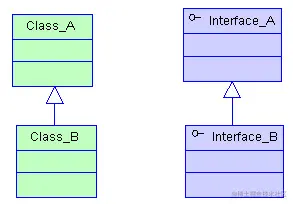``````class Animal {
name: string;

constructor(theName: string) {
this.name = theName;
}

move(distanceInMeters: number = 0) {
console.log(`\${this.name} moved \${distanceInMeters}m.`);
}
}

class Snake extends Animal {
constructor(name: string) {
super(name); // 调用父类的构造函数
}

move(distanceInMeters = 5) {
console.log("Slithering...");
super.move(distanceInMeters);
}
}

let sam = new Snake("Sammy the Python");
sam.move();
``````

#### 11.5 抽象类

``````abstract class Person {
constructor(public name: string){}

abstract say(words: string) :void;
}

// Cannot create an instance of an abstract class.(2511)
const lolo = new Person(); // Error
``````

``````abstract class Person {
constructor(public name: string){}

// 抽象方法
abstract say(words: string) :void;
}

class Developer extends Person {
constructor(name: string) {
super(name);
}

say(words: string): void {
console.log(`\${this.name} says \${words}`);
}
}

const lolo = new Developer("lolo");
lolo.say("I love ts!"); // lolo says I love ts!
``````

#### 11.6 类方法重载

``````class ProductService {
getProducts(): void;
getProducts(id: number): void;
getProducts(id?: number) {
if(typeof id === 'number') {
console.log(`获取id为 \${id} 的产品信息`);
} else {
console.log(`获取所有的产品信息`);
}
}
}

const productService = new ProductService();
productService.getProducts(666); // 获取id为 666 的产品信息
productService.getProducts(); // 获取所有的产品信息
``````

### 十二、TypeScript 泛型

#### 12.1 泛型语法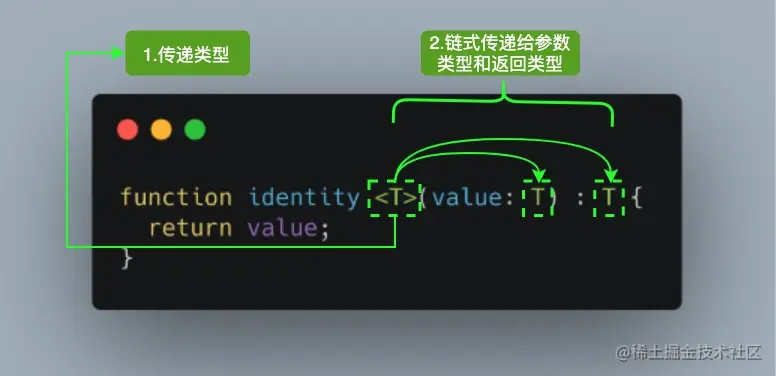• K（Key）：表示对象中的键类型；
• V（Value）：表示对象中的值类型；
• E（Element）：表示元素类型。

``````function identity <T, U>(value: T, message: U) : T {
console.log(message);
return value;
}

``````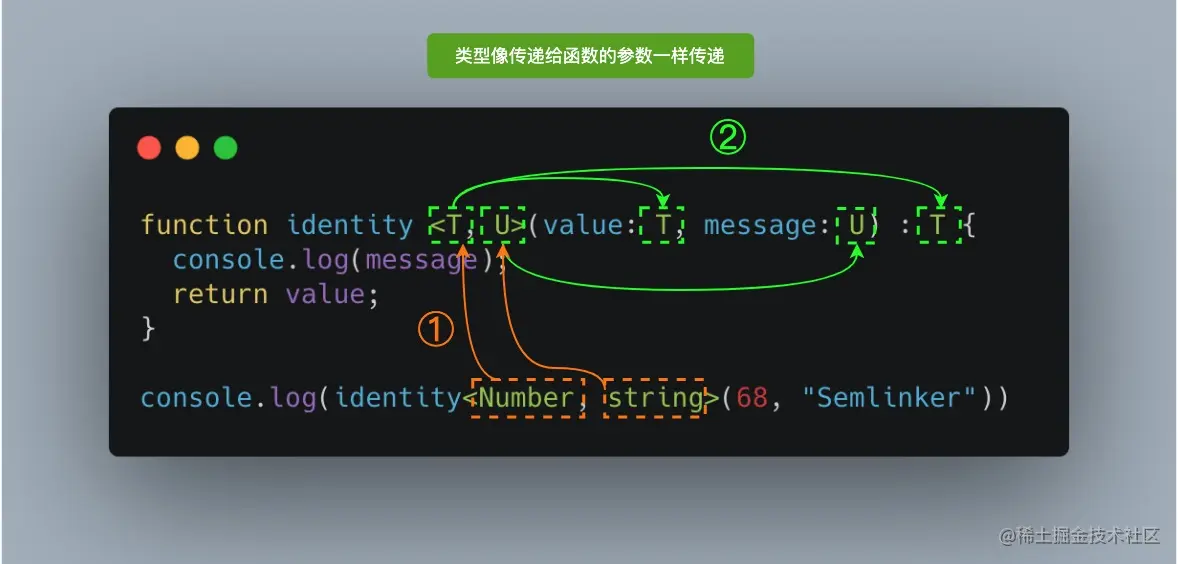``````function identity <T, U>(value: T, message: U) : T {
console.log(message);
return value;
}

``````

#### 12.2 泛型接口

``````interface GenericIdentityFn<T> {
(arg: T): T;
}
``````

#### 12.3 泛型类

``````class GenericNumber<T> {
zeroValue: T;
add: (x: T, y: T) => T;
}

let myGenericNumber = new GenericNumber<number>();
myGenericNumber.zeroValue = 0;
myGenericNumber.add = function (x, y) {
return x + y;
};
``````

#### 12.4 泛型工具类型

##### 1.typeof

``````interface Person {
name: string;
age: number;
}

const sem: Person = { name: 'semlinker', age: 33 };
type Sem= typeof sem; // -> Person

function toArray(x: number): Array<number> {
return [x];
}

type Func = typeof toArray; // -> (x: number) => number[]
``````
##### 2.keyof

`keyof` 操作符是在 TypeScript 2.1 版本引入的，该操作符可以用于获取某种类型的所有键，其返回类型是联合类型。

``````interface Person {
name: string;
age: number;
}

type K1 = keyof Person; // "name" | "age"
type K2 = keyof Person[]; // "length" | "toString" | "pop" | "push" | "concat" | "join"
type K3 = keyof { [x: string]: Person };  // string | number
``````

``````interface StringArray {
// 字符串索引 -> keyof StringArray => string | number
[index: string]: string;
}

interface StringArray1 {
// 数字索引 -> keyof StringArray1 => number
[index: number]: string;
}
``````

##### 3.in

`in` 用来遍历枚举类型：

``````type Keys = "a" | "b" | "c"

type Obj =  {
[p in Keys]: any
} // -> { a: any, b: any, c: any }
``````
##### 4.infer

``````type ReturnType<T> = T extends (
...args: any[]
) => infer R ? R : any;
``````

##### 5.extends

``````interface Lengthwise {
length: number;
}

function loggingIdentity<T extends Lengthwise>(arg: T): T {
console.log(arg.length);
return arg;
}
``````

``````loggingIdentity(3);  // Error, number doesn't have a .length property
``````

``````loggingIdentity({length: 10, value: 3});
``````
##### 6.Partial

`Partial<T>` 的作用就是将某个类型里的属性全部变为可选项 `?`

``````/**
* node_modules/typescript/lib/lib.es5.d.ts
* Make all properties in T optional
*/
type Partial<T> = {
[P in keyof T]?: T[P];
};
``````

``````interface Todo {
title: string;
description: string;
}

function updateTodo(todo: Todo, fieldsToUpdate: Partial<Todo>) {
return { ...todo, ...fieldsToUpdate };
}

const todo1 = {
title: "Learn TS",
description: "Learn TypeScript",
};

const todo2 = updateTodo(todo1, {
description: "Learn TypeScript Enum",
});
``````

``````{
title?: string | undefined;
description?: string | undefined;
}
``````

### 十三、TypeScript 装饰器

#### 13.1 装饰器是什么

• 它是一个表达式
• 该表达式被执行后，返回一个函数
• 函数的入参分别为 target、name 和 descriptor
• 执行该函数后，可能返回 descriptor 对象，用于配置 target 对象

#### 13.2 装饰器的分类

• 类装饰器（Class decorators）
• 属性装饰器（Property decorators）
• 方法装饰器（Method decorators）
• 参数装饰器（Parameter decorators）

``````tsc --target ES5 --experimentalDecorators
``````

tsconfig.json

``````{
"compilerOptions": {
"target": "ES5",
"experimentalDecorators": true
}
}
``````

#### 13.3 类装饰器

``````declare type ClassDecorator = <TFunction extends Function>(
target: TFunction
) => TFunction | void;
``````

• target: TFunction - 被装饰的类

``````function Greeter(target: Function): void {
target.prototype.greet = function (): void {
};
}

@Greeter
class Greeting {
constructor() {
// 内部实现
}
}

let myGreeting = new Greeting();
(myGreeting as any).greet(); // console output: 'Hello Semlinker!';
``````

``````function Greeter(greeting: string) {
return function (target: Function) {
target.prototype.greet = function (): void {
console.log(greeting);
};
};
}

@Greeter("Hello TS!")
class Greeting {
constructor() {
// 内部实现
}
}

let myGreeting = new Greeting();
(myGreeting as any).greet(); // console output: 'Hello TS!';
``````

#### 13.4 属性装饰器

``````declare type PropertyDecorator = (target:Object,
propertyKey: string | symbol ) => void;
``````

• target: Object - 被装饰的类
• propertyKey: string | symbol - 被装饰类的属性名

``````function logProperty(target: any, key: string) {
delete target[key];

const backingField = "_" + key;

Object.defineProperty(target, backingField, {
writable: true,
enumerable: true,
configurable: true
});

// property getter
const getter = function (this: any) {
const currVal = this[backingField];
console.log(`Get: \${key} => \${currVal}`);
return currVal;
};

// property setter
const setter = function (this: any, newVal: any) {
console.log(`Set: \${key} => \${newVal}`);
this[backingField] = newVal;
};

// Create new property with getter and setter
Object.defineProperty(target, key, {
get: getter,
set: setter,
enumerable: true,
configurable: true
});
}

class Person {
@logProperty
public name: string;

constructor(name : string) {
this.name = name;
}
}

p1.name = "kakuqo";
``````

``````Set: name => semlinker
Set: name => kakuqo
``````

#### 13.5 方法装饰器

``````declare type MethodDecorator = <T>(target:Object, propertyKey: string | symbol,
descriptor: TypePropertyDescript<T>) => TypedPropertyDescriptor<T> | void;
``````

• target: Object - 被装饰的类
• propertyKey: string | symbol - 方法名
• descriptor: TypePropertyDescript - 属性描述符

``````function log(target: Object, propertyKey: string, descriptor: PropertyDescriptor) {
let originalMethod = descriptor.value;
descriptor.value = function (...args: any[]) {
console.log("wrapped function: before invoking " + propertyKey);
let result = originalMethod.apply(this, args);
console.log("wrapped function: after invoking " + propertyKey);
return result;
};
}

@log
console.log("runTask invoked, args: " + arg);
return "finished";
}
}

console.log("result: " + result);
``````

``````"wrapped function: before invoking runTask"
"result: finished"
``````

#### 13.6 参数装饰器

``````declare type ParameterDecorator = (target: Object, propertyKey: string | symbol,
parameterIndex: number ) => void
``````

• target: Object - 被装饰的类
• propertyKey: string | symbol - 方法名
• parameterIndex: number - 方法中参数的索引值
``````function Log(target: Function, key: string, parameterIndex: number) {
let functionLogged = key || target.prototype.constructor.name;
console.log(`The parameter in position \${parameterIndex} at \${functionLogged} has
been decorated`);
}

class Greeter {
greeting: string;
constructor(@Log phrase: string) {
this.greeting = phrase;
}
}
``````

``````"The parameter in position 0 at Greeter has been decorated"
``````

### 十四、TypeScript 4.0 新特性

TypeScript 4.0 带来了很多新的特性，这里我们只简单介绍其中的两个新特性。

#### 14.1 构造函数的类属性推断

`noImplicitAny` 配置属性被启用之后，TypeScript 4.0 就可以使用控制流分析来确认类中的属性类型：

``````class Person {
fullName; // (property) Person.fullName: string
firstName; // (property) Person.firstName: string
lastName; // (property) Person.lastName: string

constructor(fullName: string) {
this.fullName = fullName;
this.firstName = fullName.split(" ");
this.lastName =   fullName.split(" ");
}
}
``````

``````class Person {
// Member 'fullName' implicitly has an 'any' type.(7008)
fullName; // Error
firstName; // Error
lastName; // Error

constructor(fullName: string) {
this.fullName = fullName;
this.firstName = fullName.split(" ");
this.lastName =   fullName.split(" ");
}
}
``````

``````class Person {
fullName;  // (property) Person.fullName: string
firstName; // (property) Person.firstName: string | undefined
lastName; // (property) Person.lastName: string | undefined

constructor(fullName: string) {
this.fullName = fullName;
if(Math.random()){
this.firstName = fullName.split(" ");
this.lastName =   fullName.split(" ");
}
}
}
``````

#### 14.2 标记的元组元素

``````function addPerson(...args: [string, number]): void {
console.log(`Person info: name: \${args}, age: \${args}`)
}

addPerson("lolo", 5); // Person info: name: lolo, age: 5
``````

``````function addPerson(name: string, age: number) {
console.log(`Person info: name: \${name}, age: \${age}`)
}
``````

``````function addPerson(...args: [name: string, age: number]): void {
console.log(`Person info: name: \${args}, age: \${args}`);
}
``````

``````// 未使用标签的智能提示
// addPerson(args_0: string, args_1: number): void
function addPerson(...args: [string, number]): void {
console.log(`Person info: name: \${args}, age: \${args}`)
}

// 已使用标签的智能提示
// addPerson(name: string, age: number): void
function addPerson(...args: [name: string, age: number]): void {
console.log(`Person info: name: \${args}, age: \${args}`);
}
``````

### 十五、编译上下文

#### 15.1 tsconfig.json 的作用

• 用于标识 TypeScript 项目的根路径；
• 用于配置 TypeScript 编译器；
• 用于指定编译的文件。

#### 15.2 tsconfig.json 重要字段

• files - 设置要编译的文件的名称；
• include - 设置需要进行编译的文件，支持路径模式匹配；
• exclude - 设置无需进行编译的文件，支持路径模式匹配；
• compilerOptions - 设置与编译流程相关的选项。

#### 15.3 compilerOptions 选项

compilerOptions 支持很多选项，常见的有 `baseUrl``target``baseUrl``moduleResolution``lib` 等。

compilerOptions 每个选项的详细说明如下：

``````{
"compilerOptions": {

/* 基本选项 */
"target": "es5",                       // 指定 ECMAScript 目标版本: 'ES3' (default), 'ES5', 'ES6'/'ES2015', 'ES2016', 'ES2017', or 'ESNEXT'
"module": "commonjs",                  // 指定使用模块: 'commonjs', 'amd', 'system', 'umd' or 'es2015'
"lib": [],                             // 指定要包含在编译中的库文件
"allowJs": true,                       // 允许编译 javascript 文件
"checkJs": true,                       // 报告 javascript 文件中的错误
"jsx": "preserve",                     // 指定 jsx 代码的生成: 'preserve', 'react-native', or 'react'
"declaration": true,                   // 生成相应的 '.d.ts' 文件
"sourceMap": true,                     // 生成相应的 '.map' 文件
"outFile": "./",                       // 将输出文件合并为一个文件
"outDir": "./",                        // 指定输出目录
"rootDir": "./",                       // 用来控制输出目录结构 --outDir.
"noEmit": true,                        // 不生成输出文件
"importHelpers": true,                 // 从 tslib 导入辅助工具函数
"isolatedModules": true,               // 将每个文件做为单独的模块 （与 'ts.transpileModule' 类似）.

/* 严格的类型检查选项 */
"strict": true,                        // 启用所有严格类型检查选项
"noImplicitAny": true,                 // 在表达式和声明上有隐含的 any类型时报错
"strictNullChecks": true,              // 启用严格的 null 检查
"noImplicitThis": true,                // 当 this 表达式值为 any 类型的时候，生成一个错误
"alwaysStrict": true,                  // 以严格模式检查每个模块，并在每个文件里加入 'use strict'

/* 额外的检查 */
"noUnusedLocals": true,                // 有未使用的变量时，抛出错误
"noUnusedParameters": true,            // 有未使用的参数时，抛出错误
"noImplicitReturns": true,             // 并不是所有函数里的代码都有返回值时，抛出错误
"noFallthroughCasesInSwitch": true,    // 报告 switch 语句的 fallthrough 错误。（即，不允许 switch 的 case 语句贯穿）

/* 模块解析选项 */
"moduleResolution": "node",            // 选择模块解析策略： 'node' (Node.js) or 'classic' (TypeScript pre-1.6)
"baseUrl": "./",                       // 用于解析非相对模块名称的基目录
"paths": {},                           // 模块名到基于 baseUrl 的路径映射的列表
"rootDirs": [],                        // 根文件夹列表，其组合内容表示项目运行时的结构内容
"typeRoots": [],                       // 包含类型声明的文件列表
"types": [],                           // 需要包含的类型声明文件名列表
"allowSyntheticDefaultImports": true,  // 允许从没有设置默认导出的模块中默认导入。

/* Source Map Options */
"sourceRoot": "./",                    // 指定调试器应该找到 TypeScript 文件而不是源文件的位置
"mapRoot": "./",                       // 指定调试器应该找到映射文件而不是生成文件的位置
"inlineSourceMap": true,               // 生成单个 soucemaps 文件，而不是将 sourcemaps 生成不同的文件
"inlineSources": true,                 // 将代码与 sourcemaps 生成到一个文件中，要求同时设置了 --inlineSourceMap 或 --sourceMap 属性

/* 其他选项 */
"experimentalDecorators": true,        // 启用装饰器
}
}
``````

### 十六、TypeScript 开发辅助工具

#### 16.1 TypeScript Playground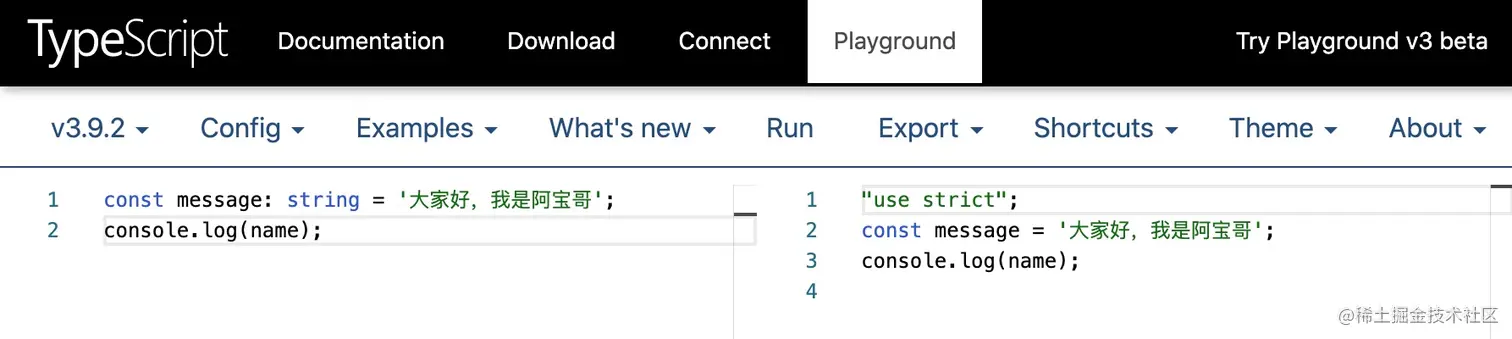#### 16.2 TypeScript UML Playground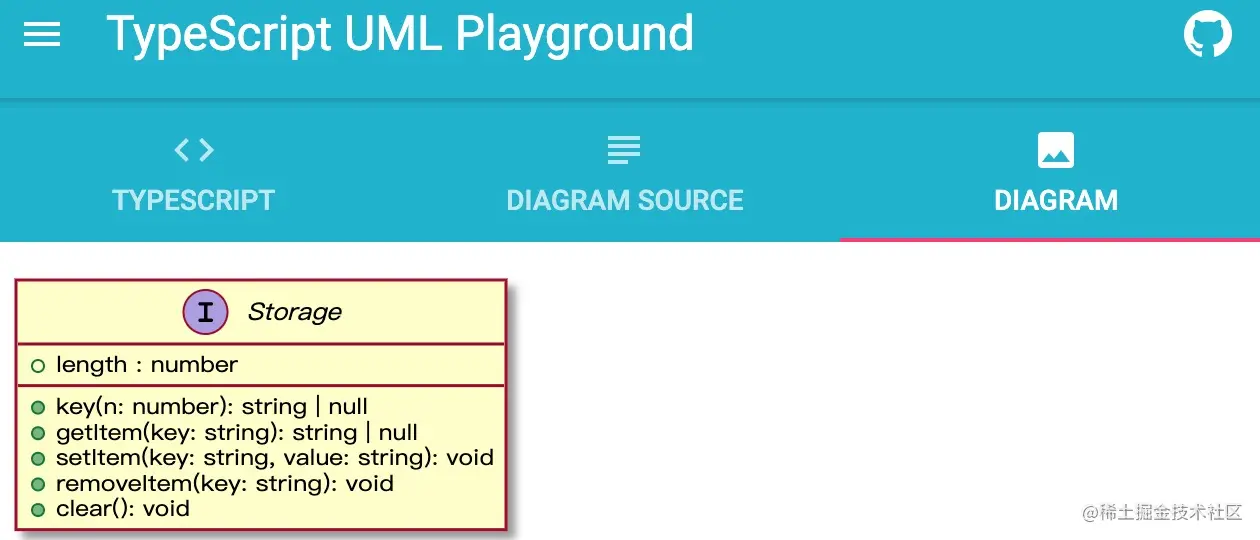#### 16.3 JSON TO TS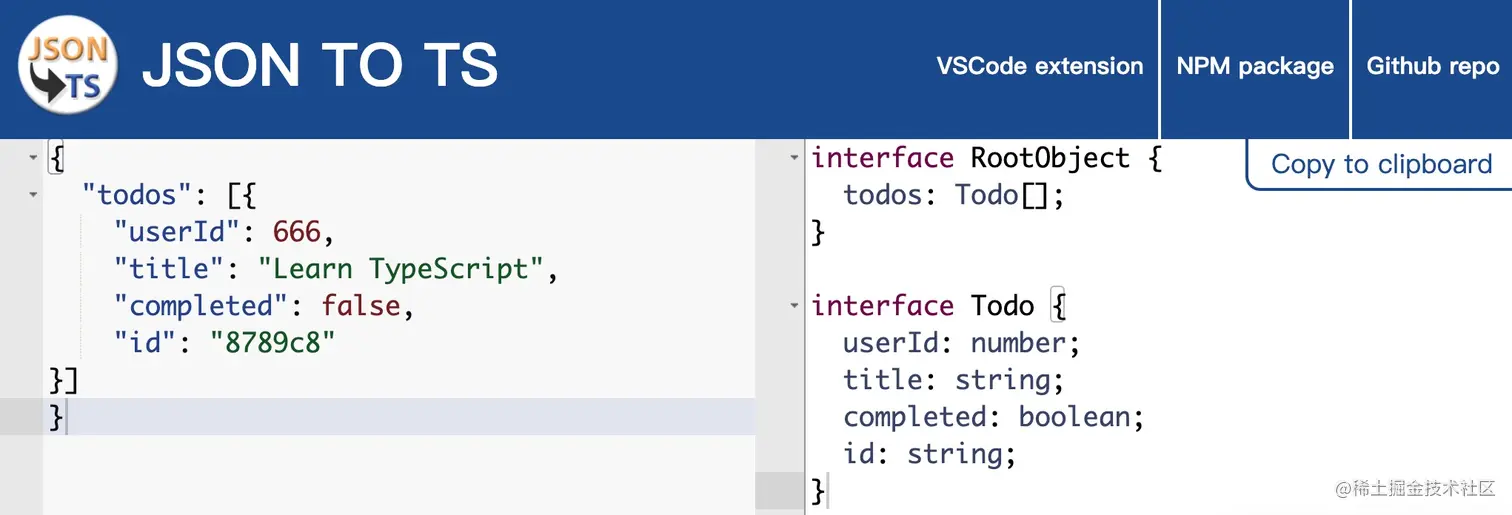#### 16.4 Schemats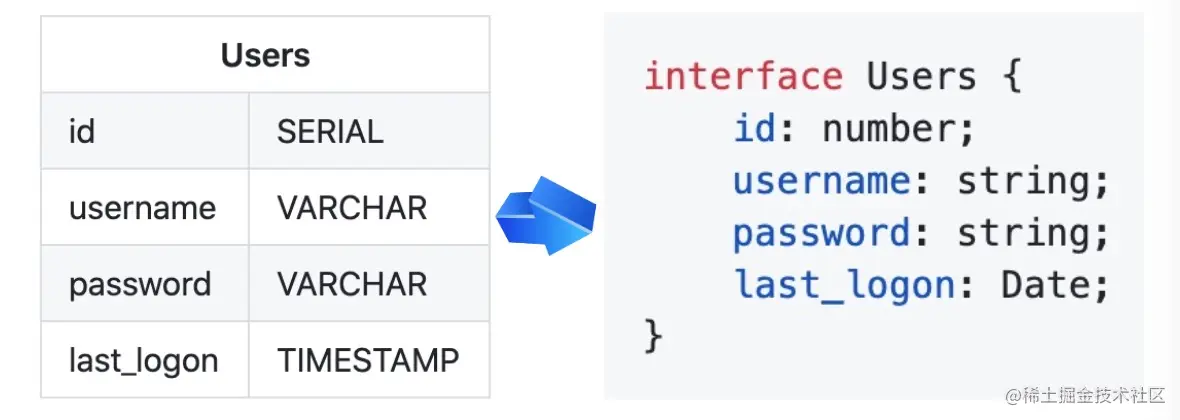#### 16.5 TypeScript AST Viewer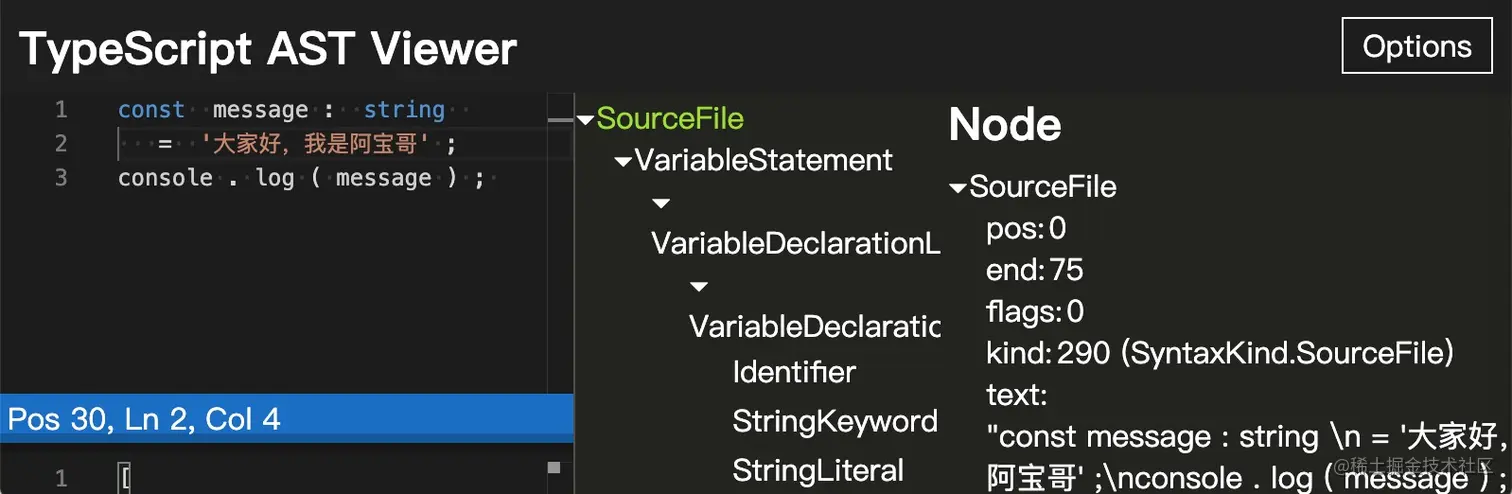#### 16.6 TypeDoc#### 16.7 TypeScript ESLintTypeScript ESLint 项目感兴趣且想在项目中应用的小伙伴，可以参考 “在Typescript项目中，如何优雅的使用ESLint和Prettier” 这篇文章。

### 十七、参考资源

•••••••••••